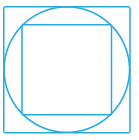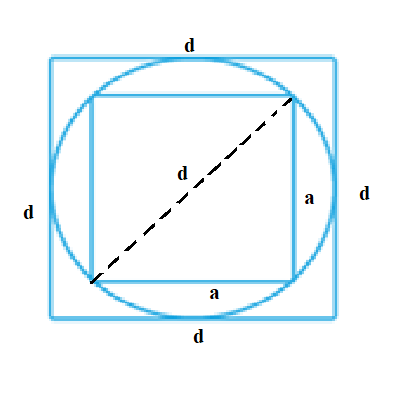#### In Figure, a square is inscribed in a circle of diameter ‘d’ and another square is circumscribing the circle. Is the area of the outer square four times the area of the inner square? Give reasons for your answer.

SolutionDiameter of circle = d

Side of biggest square = d

Area of the biggest square is = side × side

=d  d=d2

Diagonal of smallest square = d

Let side = a

d2=a2+a2                 {using Pythagoras theorem}

d2=2a2

Area of smallest square

Here we found that area of outer square is not 4 times the area of inner square.